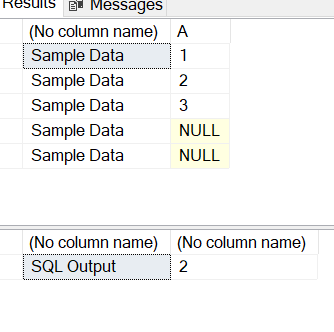# Why does CASE WHEN NULL IN (SELECT A FROM B) THEN 'Y' ELSE '' AS [NULL] does not work?

Hello

Do you have any idea why the below does not work?

CASE WHEN NULL IN (SELECT A FROM B WHERE C=D) THEN 'Y' ELSE '' END AS [NULL]

And how I can make it work?

Thanks!

I would recommend reviewing this article: https://learnsql.com/blog/understanding-use-null-sql/

To solve your problem, you can switch this up:

``````CASE WHEN (SELECT A FROM B WHERE C=D) IS NULL THEN 'Y' ELSE '' END AS [NULL]
``````

However - this can lead to another error. A sub-query used as an expression here can only return a single value. If the query returns multiple rows - or you try to return multiple columns it will fail.

NULL is never = or <> any other value. A IN (1, 2, 3, ...) is basically just a short-hand way of writing A = 1 OR A = 2 OR A = 3, so NULL will never match one of those values.

For example:
`select case when null in (select null) then 'y' else 'n' end`

Thanks but I don't think this will work because the (SELECT A FROM B WHERE C=D) usually returns a list with non-null and null values.

I managed to write what I want by doing:
CASE WHEN 0 < (SELECT COUNT(A) FROM B WHERE C=D AND E=NULL) THEN 'Y' ELSE '' END AS [NULL]

It seems to work for my case but I would have difficulties if the condition had to be A=NULL.

Any idea?

I can explain more:
If the SELECT returns a list of A as 1,NULL,2,3, I would need to identify that there is a NULL among that list.

I suppose I could do a COUNT(*) <> COUNT(A) or something to check if there is a NULL?

But I think the COUNT(*) will have to have a NULL across the whole table B ?

Hope this helps !!!Looks like you are Really New to SQL
and trying to Learn

Very Very very hard initially .. till you get the hang of a few basic thingsIf the SELECT returns a list of A as 1,NULL,2,3, I would need to identify that there is a NULL among that list.

``````drop table Table1
create table Table1
(
A int NULL
)

insert into Table1 select 1
insert into Table1 select 2
insert into Table1 select 3
insert into Table1 select NULL
insert into Table1 select NULL

select 'Sample Data',* from Table1

select 'SQL Output', sum(isnull(A,1)) from Table1 where A is null
``````1 Like